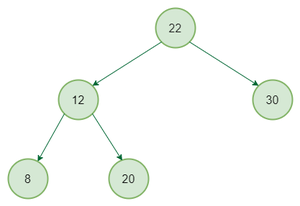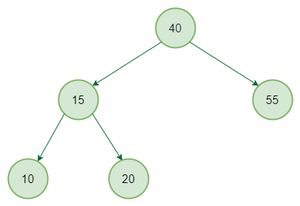Open in App
Not now

# Find the node with minimum value in a Binary Search Tree

• Difficulty Level : Basic
• Last Updated : 12 Dec, 2022

Write a function to find the node with minimum value in a Binary Search Tree.

Example:

Input:first example BST

Output: 8

Input:second example BST

Output: 10

Recommended Practice

Approach: To solve the problem follow the below idea:

This is quite simple. Just traverse the node from root to left recursively until left is NULL. The node whose left is NULL is the node with minimum value

Below is the implementation of the above approach:

## C++

 `// C++ program to find minimum value node in binary search``// Tree.``#include ``using` `namespace` `std;` `/* A binary tree node has data, pointer to left child``and a pointer to right child */``struct` `node {``    ``int` `data;``    ``struct` `node* left;``    ``struct` `node* right;``};` `/* Helper function that allocates a new node``with the given data and NULL left and right``pointers. */``struct` `node* newNode(``int` `data)``{``    ``struct` `node* node``        ``= (``struct` `node*)``malloc``(``sizeof``(``struct` `node));``    ``node->data = data;``    ``node->left = NULL;``    ``node->right = NULL;` `    ``return` `(node);``}` `/* Give a binary search tree and a number,``inserts a new node with the given number in``the correct place in the tree. Returns the new``root pointer which the caller should then use``(the standard trick to avoid using reference``parameters). */``struct` `node* insert(``struct` `node* node, ``int` `data)``{``    ``/* 1. If the tree is empty, return a new,``        ``single node */``    ``if` `(node == NULL)``        ``return` `(newNode(data));``    ``else` `{``        ``/* 2. Otherwise, recur down the tree */``        ``if` `(data <= node->data)``            ``node->left = insert(node->left, data);``        ``else``            ``node->right = insert(node->right, data);` `        ``/* return the (unchanged) node pointer */``        ``return` `node;``    ``}``}` `/* Given a non-empty binary search tree,``return the minimum data value found in that``tree. Note that the entire tree does not need``to be searched. */``int` `minValue(``struct` `node* node)``{``    ``struct` `node* current = node;` `    ``/* loop down to find the leftmost leaf */``    ``while` `(current->left != NULL) {``        ``current = current->left;``    ``}``    ``return` `(current->data);``}` `/* Driver Code*/``int` `main()``{``    ``struct` `node* root = NULL;``    ``root = insert(root, 4);``    ``insert(root, 2);``    ``insert(root, 1);``    ``insert(root, 3);``    ``insert(root, 6);``    ``insert(root, 5);` `      ``// Function call``    ``cout << ``"\n Minimum value in BST is "` `<< minValue(root);``    ``getchar``();``    ``return` `0;``}` `// This code is contributed by Mukul Singh.`

## C

 `// C program to find minimum value node in binary search``// Tree.` `#include ``#include ` `/* A binary tree node has data, pointer to left child``   ``and a pointer to right child */``struct` `node {``    ``int` `data;``    ``struct` `node* left;``    ``struct` `node* right;``};` `/* Helper function that allocates a new node``with the given data and NULL left and right``pointers. */``struct` `node* newNode(``int` `data)``{``    ``struct` `node* node``        ``= (``struct` `node*)``malloc``(``sizeof``(``struct` `node));``    ``node->data = data;``    ``node->left = NULL;``    ``node->right = NULL;` `    ``return` `(node);``}` `/* Give a binary search tree and a number,``inserts a new node with the given number in``the correct place in the tree. Returns the new``root pointer which the caller should then use``(the standard trick to avoid using reference``parameters). */``struct` `node* insert(``struct` `node* node, ``int` `data)``{``    ``/* 1. If the tree is empty, return a new,``        ``single node */``    ``if` `(node == NULL)``        ``return` `(newNode(data));``    ``else` `{``        ``/* 2. Otherwise, recur down the tree */``        ``if` `(data <= node->data)``            ``node->left = insert(node->left, data);``        ``else``            ``node->right = insert(node->right, data);` `        ``/* return the (unchanged) node pointer */``        ``return` `node;``    ``}``}` `/* Given a non-empty binary search tree,``return the minimum data value found in that``tree. Note that the entire tree does not need``to be searched. */``int` `minValue(``struct` `node* node)``{``    ``struct` `node* current = node;` `    ``/* loop down to find the leftmost leaf */``    ``while` `(current->left != NULL) {``        ``current = current->left;``    ``}``    ``return` `(current->data);``}` `/* Driver code*/``int` `main()``{``    ``struct` `node* root = NULL;``    ``root = insert(root, 4);``    ``insert(root, 2);``    ``insert(root, 1);``    ``insert(root, 3);``    ``insert(root, 6);``    ``insert(root, 5);` `      ``// Function call``    ``printf``(``"\n Minimum value in BST is %d"``, minValue(root));``    ``getchar``();``    ``return` `0;``}`

## Java

 `// Java program to find minimum value node in Binary Search``// Tree` `// A binary tree node``class` `Node {` `    ``int` `data;``    ``Node left, right;` `    ``Node(``int` `d)``    ``{``        ``data = d;``        ``left = right = ``null``;``    ``}``}` `class` `BinaryTree {` `    ``static` `Node head;` `    ``/* Given a binary search tree and a number,``     ``inserts a new node with the given number in``     ``the correct place in the tree. Returns the new``     ``root pointer which the caller should then use``     ``(the standard trick to avoid using reference``     ``parameters). */``    ``Node insert(Node node, ``int` `data)``    ``{` `        ``/* 1. If the tree is empty, return a new,``         ``single node */``        ``if` `(node == ``null``) {``            ``return` `(``new` `Node(data));``        ``}``        ``else` `{` `            ``/* 2. Otherwise, recur down the tree */``            ``if` `(data <= node.data) {``                ``node.left = insert(node.left, data);``            ``}``            ``else` `{``                ``node.right = insert(node.right, data);``            ``}` `            ``/* return the (unchanged) node pointer */``            ``return` `node;``        ``}``    ``}` `    ``/* Given a non-empty binary search tree,``     ``return the minimum data value found in that``     ``tree. Note that the entire tree does not need``     ``to be searched. */``    ``int` `minvalue(Node node)``    ``{``        ``Node current = node;` `        ``/* loop down to find the leftmost leaf */``        ``while` `(current.left != ``null``) {``            ``current = current.left;``        ``}``        ``return` `(current.data);``    ``}` `    ``// Driver code``    ``public` `static` `void` `main(String[] args)``    ``{``        ``BinaryTree tree = ``new` `BinaryTree();``        ``Node root = ``null``;``        ``root = tree.insert(root, ``4``);``        ``tree.insert(root, ``2``);``        ``tree.insert(root, ``1``);``        ``tree.insert(root, ``3``);``        ``tree.insert(root, ``6``);``        ``tree.insert(root, ``5``);` `          ``// Function call``        ``System.out.println(``"Minimum value of BST is "``                           ``+ tree.minvalue(root));``    ``}``}` `// This code is contributed by Mayank Jaiswal`

## Python3

 `# Python3 program to find the node with minimum value in bst` `# A binary tree node`  `class` `Node:` `    ``# Constructor to create a new node``    ``def` `__init__(``self``, key):``        ``self``.data ``=` `key``        ``self``.left ``=` `None``        ``self``.right ``=` `None`  `""" Give a binary search tree and a number,``inserts a new node with the given number in``the correct place in the tree. Returns the new``root pointer which the caller should then use``(the standard trick to avoid using reference``parameters). """`  `def` `insert(node, data):` `    ``# 1. If the tree is empty, return a new,``    ``# single node``    ``if` `node ``is` `None``:``        ``return` `(Node(data))` `    ``else``:``        ``# 2. Otherwise, recur down the tree``        ``if` `data <``=` `node.data:``            ``node.left ``=` `insert(node.left, data)``        ``else``:``            ``node.right ``=` `insert(node.right, data)` `        ``# Return the (unchanged) node pointer``        ``return` `node`  `""" Given a non-empty binary search tree, ``return the minimum data value found in that``tree. Note that the entire tree does not need``to be searched. """`  `def` `minValue(node):``    ``current ``=` `node` `    ``# loop down to find the leftmost leaf``    ``while``(current.left ``is` `not` `None``):``        ``current ``=` `current.left` `    ``return` `current.data`  `# Driver code``if` `__name__ ``=``=` `'__main__'``:``  ``root ``=` `None``  ``root ``=` `insert(root, ``4``)``  ``insert(root, ``2``)``  ``insert(root, ``1``)``  ``insert(root, ``3``)``  ``insert(root, ``6``)``  ``insert(root, ``5``)` `  ``# Function call``  ``print``(``"\nMinimum value in BST is %d"` `%` `(minValue(root)))` `# This code is contributed by Nikhil Kumar Singh(nickzuck_007)`

## C#

 `// C# program to find minimum value node in Binary Search``// Tree` `using` `System;` `// C# program to find minimum value node in Binary Search``// Tree` `// A binary tree node``public` `class` `Node {` `    ``public` `int` `data;``    ``public` `Node left, right;` `    ``public` `Node(``int` `d)``    ``{``        ``data = d;``        ``left = right = ``null``;``    ``}``}` `public` `class` `BinaryTree {` `    ``public` `static` `Node head;` `    ``/* Given a binary search tree and a number,``     ``inserts a new node with the given number in``     ``the correct place in the tree. Returns the new``     ``root pointer which the caller should then use``     ``(the standard trick to avoid using reference``     ``parameters). */``    ``public` `virtual` `Node insert(Node node, ``int` `data)``    ``{` `        ``/* 1. If the tree is empty, return a new,``         ``single node */``        ``if` `(node == ``null``) {``            ``return` `(``new` `Node(data));``        ``}``        ``else` `{` `            ``/* 2. Otherwise, recur down the tree */``            ``if` `(data <= node.data) {``                ``node.left = insert(node.left, data);``            ``}``            ``else` `{``                ``node.right = insert(node.right, data);``            ``}` `            ``/* return the (unchanged) node pointer */``            ``return` `node;``        ``}``    ``}` `    ``/* Given a non-empty binary search tree,``     ``return the minimum data value found in that``     ``tree. Note that the entire tree does not need``     ``to be searched. */``    ``public` `virtual` `int` `minvalue(Node node)``    ``{``        ``Node current = node;` `        ``/* loop down to find the leftmost leaf */``        ``while` `(current.left != ``null``) {``            ``current = current.left;``        ``}``        ``return` `(current.data);``    ``}` `    ``// Driver code``    ``public` `static` `void` `Main(``string``[] args)``    ``{``        ``BinaryTree tree = ``new` `BinaryTree();``        ``Node root = ``null``;``        ``root = tree.insert(root, 4);``        ``tree.insert(root, 2);``        ``tree.insert(root, 1);``        ``tree.insert(root, 3);``        ``tree.insert(root, 6);``        ``tree.insert(root, 5);` `          ``// Function call``        ``Console.WriteLine(``"Minimum value of BST is "``                          ``+ tree.minvalue(root));``    ``}``}` `// This code is contributed by Shrikant13`

## PHP

 `node = ``\$node``;``        ``\$left` `= ``\$right` `= NULL;``    ``}` `    ``// set the left node in tree``    ``function` `set_left(``\$left``)``    ``{``        ``\$this``->left = ``\$left``;``    ``}` `    ``// set the right node in tree``    ``function` `set_right(``\$right``)``    ``{``        ``\$this``->right = ``\$right``;``    ``}` `    ``// get left node``    ``function` `get_left()``    ``{``        ``return` `\$this``->left;``    ``}` `    ``// get right node``    ``function` `get_right()``    ``{``        ``return` `\$this``->right;``    ``}` `    ``// get value of current node``    ``function` `get_node()``    ``{``        ``return` `\$this``->node;``    ``}``    ` `}` `// Find the node with minimum value``// in a Binary Search Tree``function` `get_minimum_value(``\$node``)``{``    ``/*travel till last left node to``      ``get the minimum value*/``    ``while` `(``\$node``->get_left() != NULL)``    ``{``        ``\$node` `= ``\$node``->get_left();``    ``}``    ``return` `\$node``->get_node();``}` `// code to creating a tree``\$node` `= ``new` `node(4);``\$lnode` `= ``new` `node(2);``\$lnode``->set_left(``new` `node(1));``\$lnode``->set_right(``new` `node(3));``\$rnode` `= ``new` `node(6);``\$rnode``->set_left(``new` `node(5));``\$node``->set_left(``\$lnode``);``\$node``->set_right(``\$rnode``);` `\$minimum_value` `= get_minimum_value(``\$node``);``echo` `'Minimum value of BST is '``.``                 ``\$minimum_value``;` `// This code is contributed``// by Deepika Pathak``?>`

## Javascript

 ``

Output

` Minimum value in BST is 1`

Time Complexity: O(Height of the BST)
Auxiliary Space: O(1)

My Personal Notes arrow_drop_up